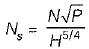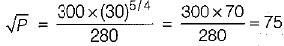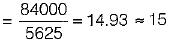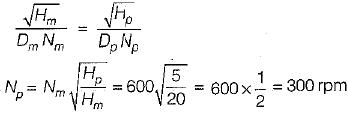Courses

# Hydroelectric Power Plant - 2

## 10 Questions MCQ Test Topicwise Question Bank for GATE Mechanical Engineering | Hydroelectric Power Plant - 2

Description
This mock test of Hydroelectric Power Plant - 2 for Mechanical Engineering helps you for every Mechanical Engineering entrance exam. This contains 10 Multiple Choice Questions for Mechanical Engineering Hydroelectric Power Plant - 2 (mcq) to study with solutions a complete question bank. The solved questions answers in this Hydroelectric Power Plant - 2 quiz give you a good mix of easy questions and tough questions. Mechanical Engineering students definitely take this Hydroelectric Power Plant - 2 exercise for a better result in the exam. You can find other Hydroelectric Power Plant - 2 extra questions, long questions & short questions for Mechanical Engineering on EduRev as well by searching above.
QUESTION: 1

Solution:
QUESTION: 2

Solution:
QUESTION: 3

### The available discharge and head of a proposed hydroelectric power plant are 350 m3/s and 30 m respectively. The turbine efficiency is 80% and speed is 280 rpm. What is the least number of machines required if you have to use Francsis turbine having a specific speed of 300? [Take g = 10 m/s2 and 305/4 = 70]

Solution:

P = g Q Hη
= 10 x 350 x 30 x 0.8 = 84000 kW
Specific speed,∴∴ P = 752 = 5625 kW
∴ Number of turbineQUESTION: 4

A turbine works under a head of 20 m and has maximum flow rate of 3 m3/s and a speed of 600 rpm. If it has to work in another plant under a head of 5 m, at what speed must the turbine run in order to attain approximately the same efficiency

Solution:QUESTION: 5

The speed factor (speed ratio) of a Pelton turbine for its efficient operation lies in the range

Solution:
QUESTION: 6

Regulation of a pelton turbine is done by changing

Solution:

A spear or needle is so arranged that it can move forward or backward thereby decreasing or increasing the annular area of the nozzle passage. This spear nozzle regulates the water flow through the nozzle.

QUESTION: 7

The movable wicket gates of a reaction turbine are used to

Solution:

Guide vanes or wicket gates are arranged inside the casing to form a number of flow passages between the casing and runner blades. They direct the water onto the runner at an angle appropriate to the design.

QUESTION: 8

Which one of the following forms of draft tube will not improve the hydraulic efficiency of the turbine?

Solution:

Purpose of draft tube is to convert kinetic energy into pressure energy, so option (a) wiil not be suitable for the intended purpose of draft tube.

QUESTION: 9

The value of speed ratio for a Kaplan turbine is about

Solution:
QUESTION: 10

The specific speed of a fluid machine

Solution: